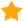7 doctors weighed in:

How accurate is the body mass index?

7 doctors weighed in
6 doctors agree

In brief: Mathematical

It is a specific formula to calculate the bmi, based on one's own height and weight.
Bmi = weight (kg) divided by height (m) squared.

In brief: Mathematical

It is a specific formula to calculate the bmi, based on one's own height and weight.
Bmi = weight (kg) divided by height (m) squared.
Dr. Mark Hoepfner
Dr. Mark Hoepfner
Get help from a real doctor now
Dr. Cindy Juster
Board Certified, Pediatrics
34 years in practice
2M people helped111,000 doctors available